## 2.2 Describe the data

The different representations are done with the plot function.

### 2.2.1 unipart network on seed lots

For network representation, set plot_type = "network" diffusion event are displayed with a curve. in_col can be settled to customize color of vertex.

p_net = plot(net_unipart_sl, plot_type = "network", in_col = "location")
p_net
## []
## []$network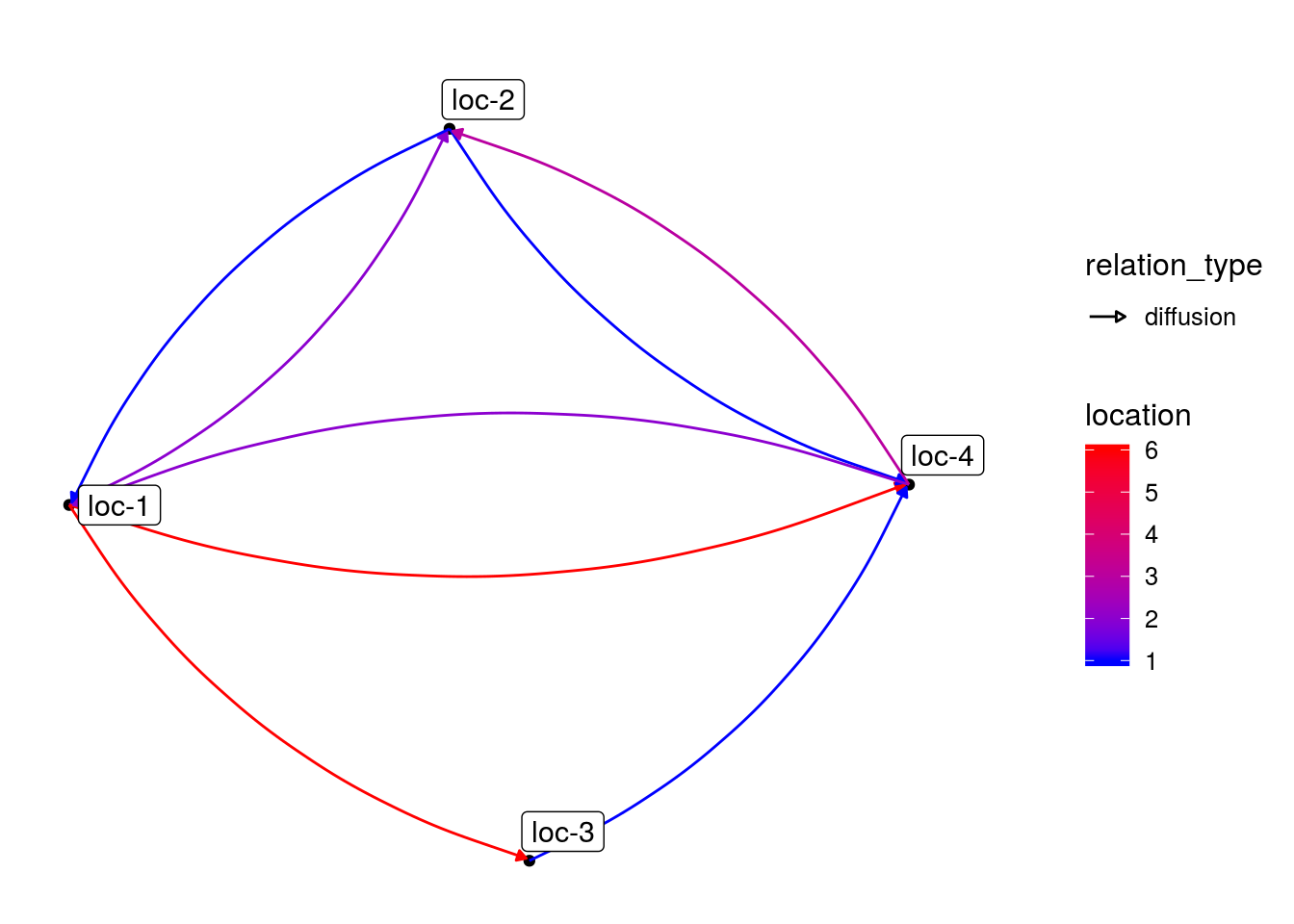In order to get the network organized in a chronologiical order and by location, set organize_sl = TRUE. This representation is possible if the seed lots are under the following format : GERMPLASM_LOCATION_YEAR_DIGIT. p_net_org = plot(net_unipart_sl, plot_type = "network", organize_sl = TRUE) p_net_org ## [] ## []$network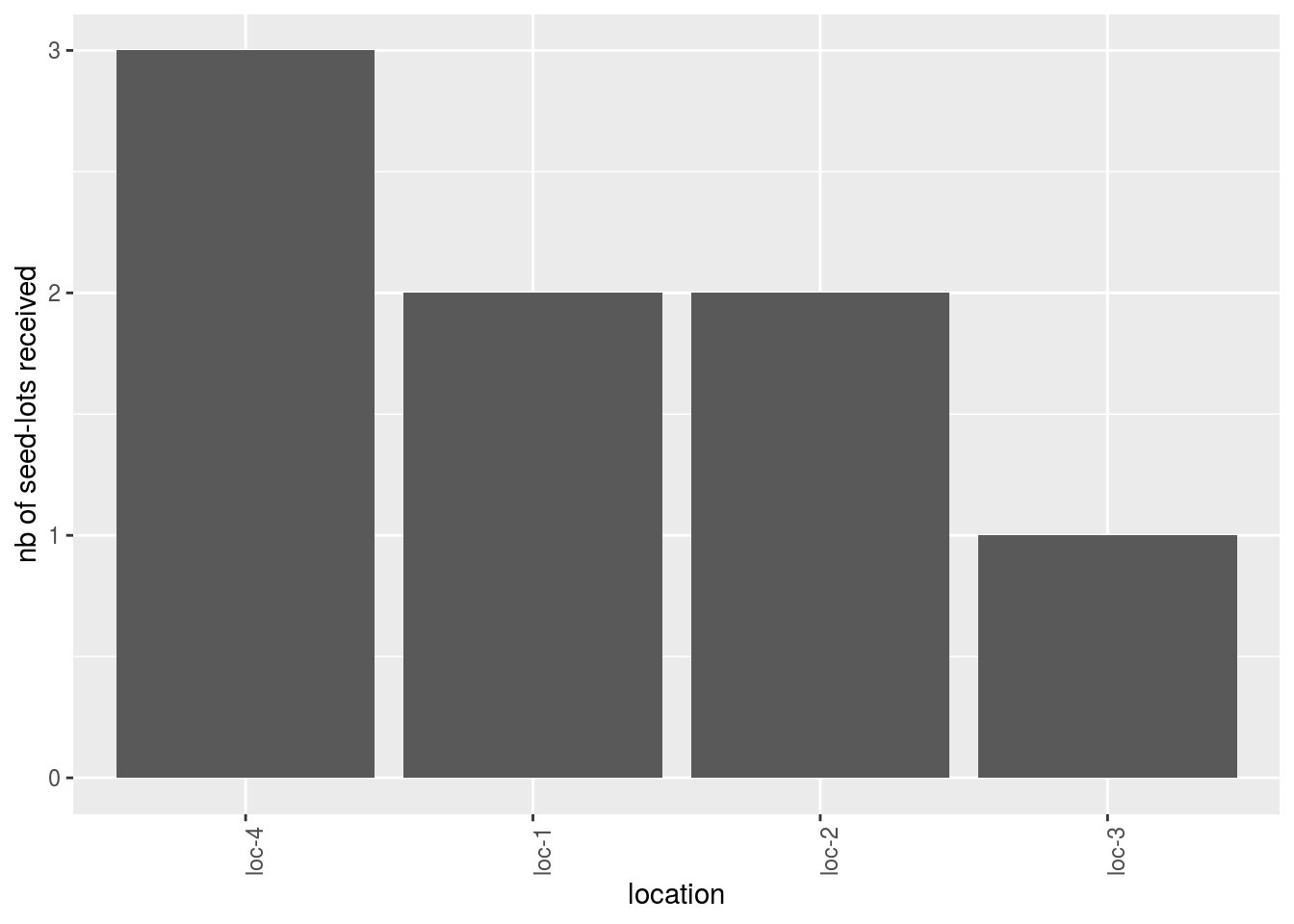To have information on the seed lots that are represented, plot_type = "barplot" can be used. Choose what to represent on the x axis and in color as well as the number of parameter per plot.

p_bar = plot(net_unipart_sl, plot_type = "barplot", in_col = "location",
x_axis = "germplasm", nb_parameters_per_plot_x_axis = 5,
nb_parameters_per_plot_in_col = 5)
p_bar[]$barplot$germplasm-1|location-1 # first element of the plot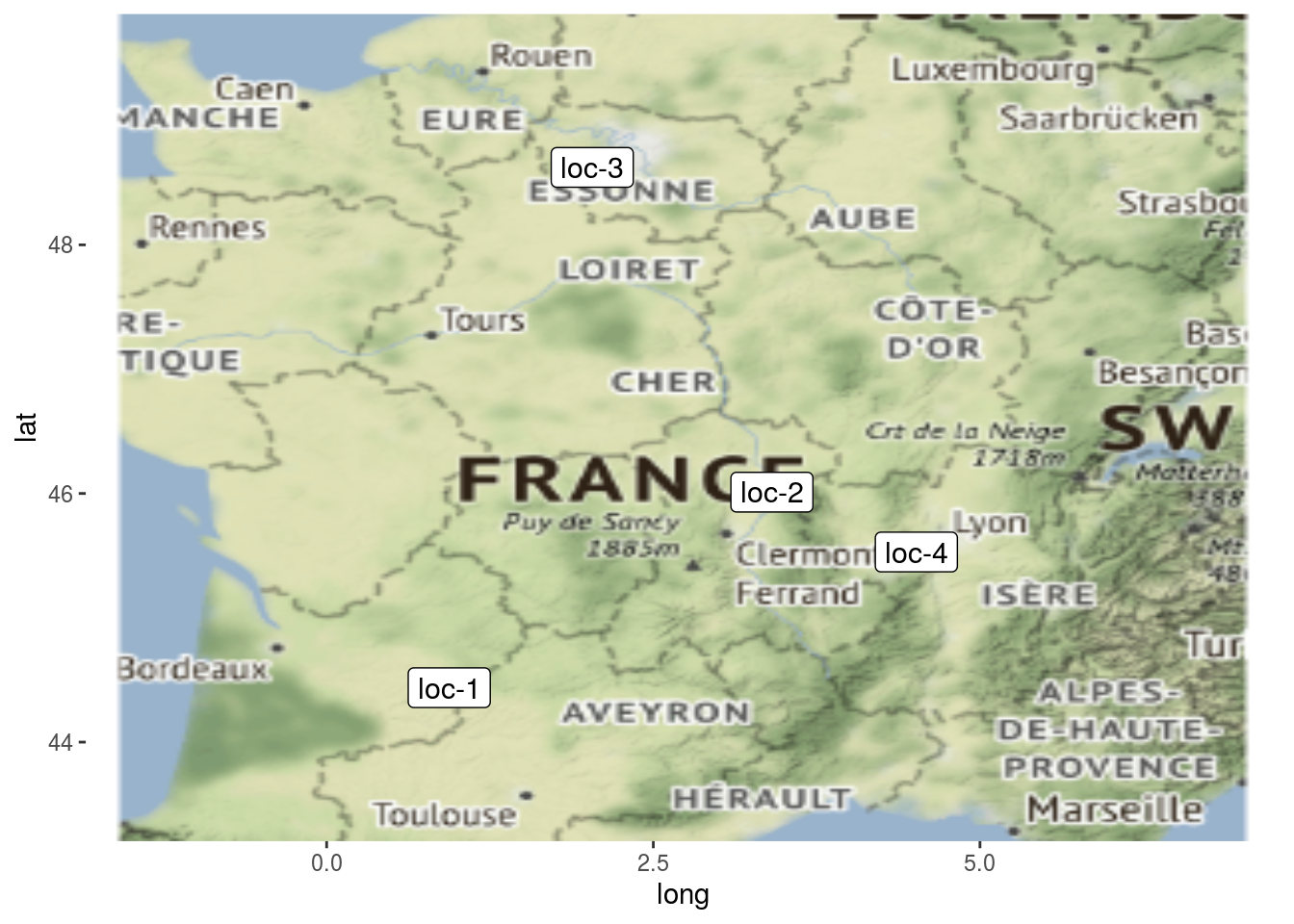Barplot can also be use to study the relation within the network. The name of the relation must be put in the argument vec_variables. The results is a list of two elements for each variable:

• nb_received: number of seed lots that end the relation
• nb_given: number of seed lots that start the relation
p_bar = plot(net_unipart_sl, plot_type = "barplot", vec_variables = "diffusion",
nb_parameters_per_plot_x_axis = 100, x_axis = "location", in_col = "year")
p_bar
## []
## []$diffusion ## []$diffusion$nb_received ## []$diffusion$nb_received$location-1|year-1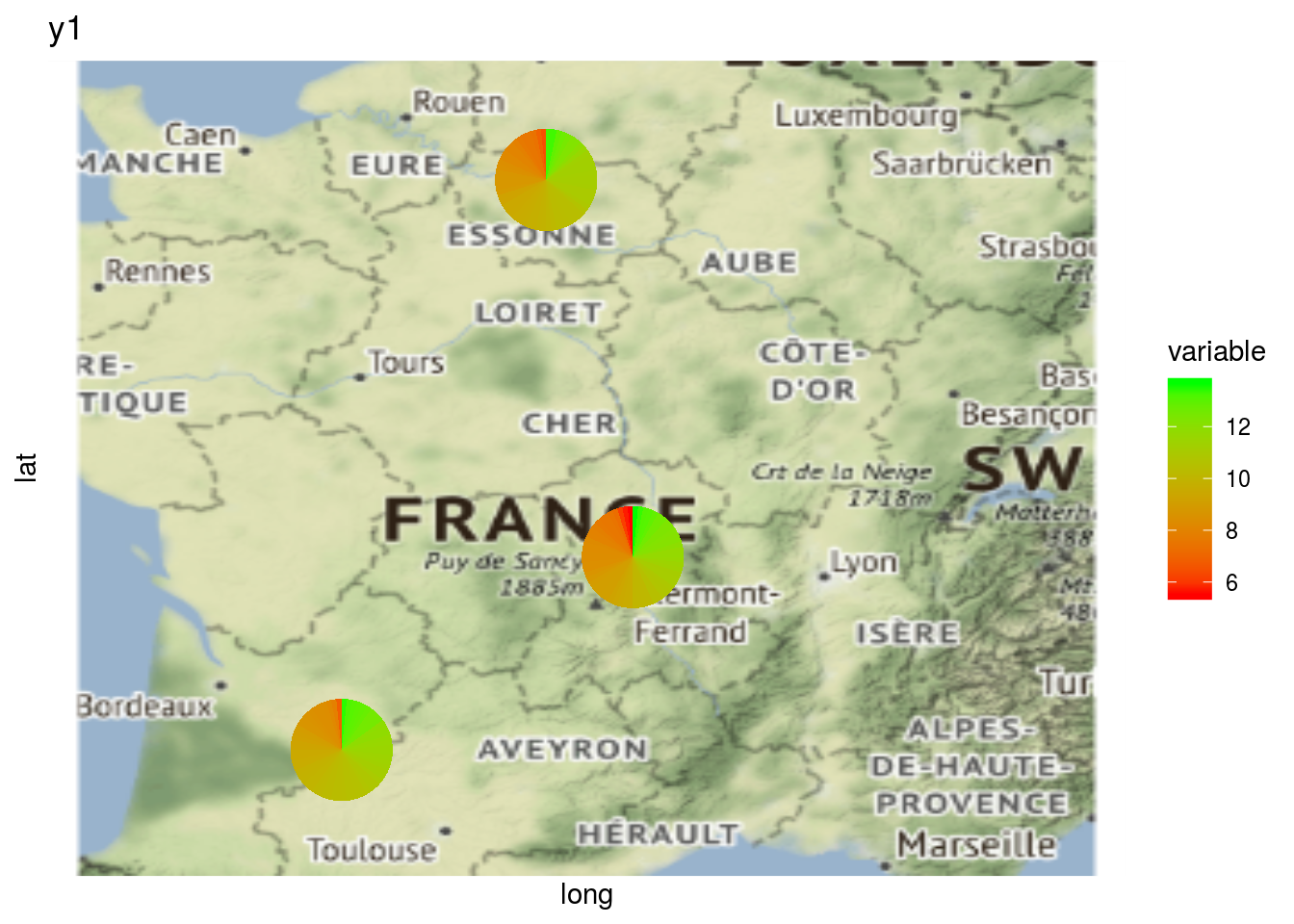##
##
## []$diffusion$nb_given
## []$diffusion$nb_given$location-1|year-1Location present on the network can be displayed on a map with plot_type = "map" p_map = plot(net_unipart_sl, plot_type = "map", labels_on = "location") p_map ## [] ## []$map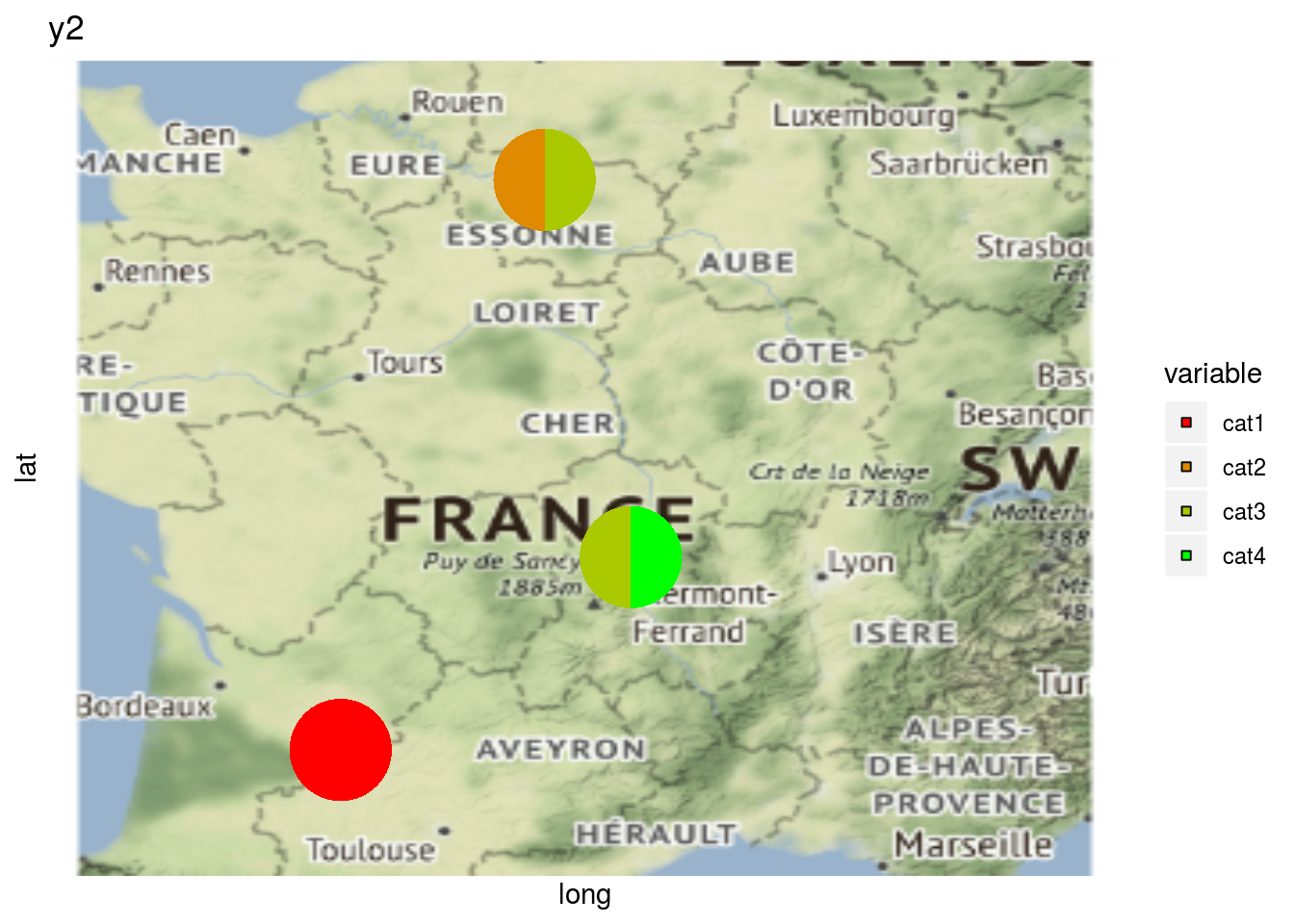It can be interesting to plot information regarding a variable on map with a pie with plot_type = "map" and by setting arguments data_to_pie and variable:

nb_values = 30
data_to_pie = data.frame( # = data_agro : ajouter id_seed_lot dans les format agro
id = rep(c("germ-4_loc-4_2009_0001", "germ-9_loc-4_2009_0001", "germ-10_loc-3_2009_0001", "germ-12_loc-3_2007_0001", "germ-11_loc-2_2009_0001", "germ-10_loc-2_2009_0001"), each = nb_values),
location = rep(c("loc-1", "loc-1", "loc-3", "loc-3", "loc-2", "loc-2"), each = nb_values),
year = rep(c("2009", "2008", "2007", "2007", "2009", "2009"), each = nb_values),
germplasm = rep(c("germ-7", "germ-2", "germ-6", "germ-4", "germ-5", "germ-13"), each = nb_values),
block = 1,
X = 1,
Y = 1,
y1 = rnorm(nb_values*6, 10, 2), # quanti
y2 = rep(c("cat1", "cat1", "cat2", "cat3", "cat3", "cat4"), each = nb_values)  # quali
)
# y1 is a quantitative variable
p_map_pies_y1 = plot(net_unipart_sl, data_to_pie, plot_type = "map", vec_variables = "y1")
p_map_pies_y1
## []
## []$y1_map_with_pies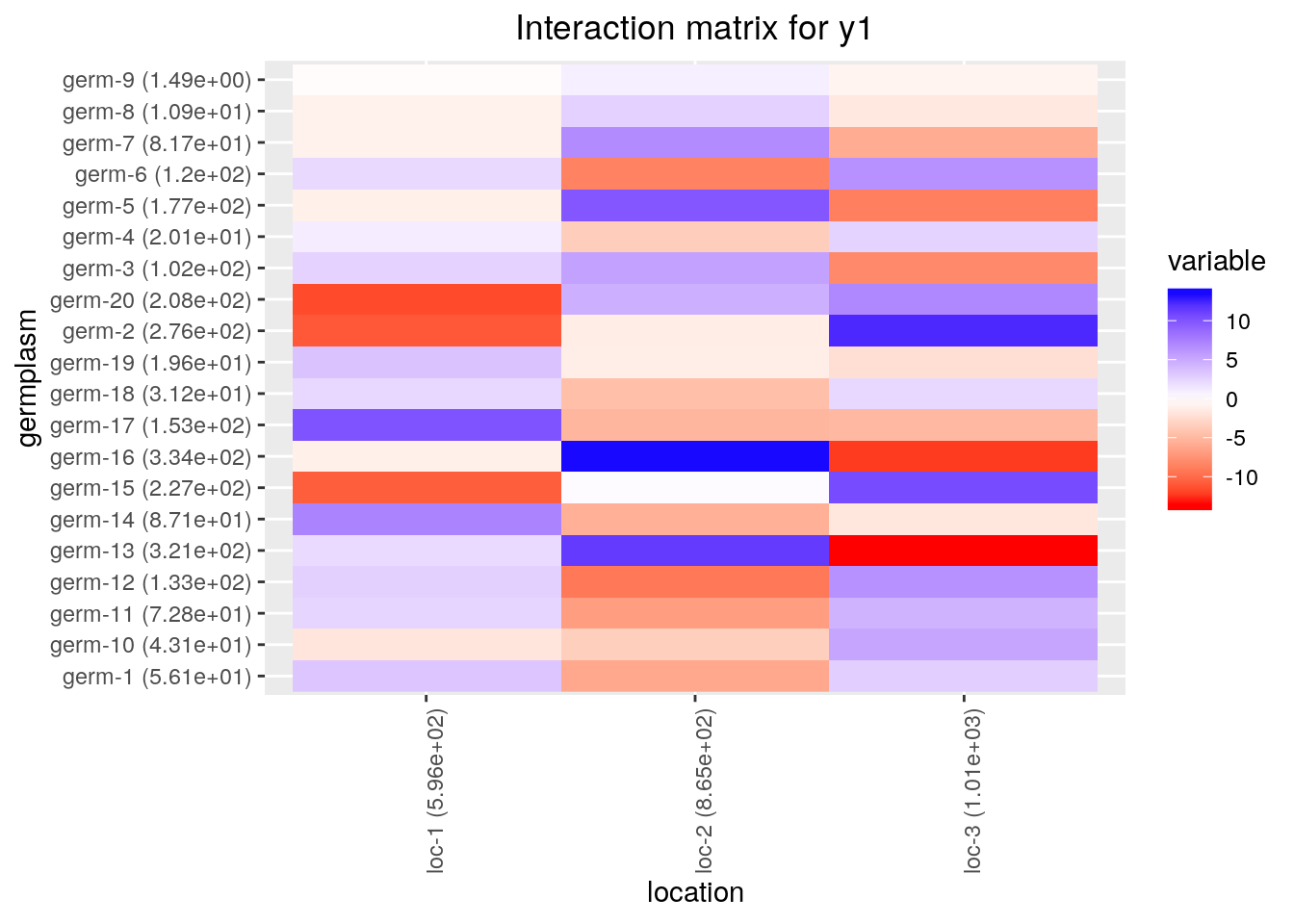# y2 is a qualitative variable p_map_pies_y2 = plot(net_unipart_sl, data_to_pie, plot_type = "map", vec_variables = "y2") p_map_pies_y2 ## [] ## []$y2_map_with_pies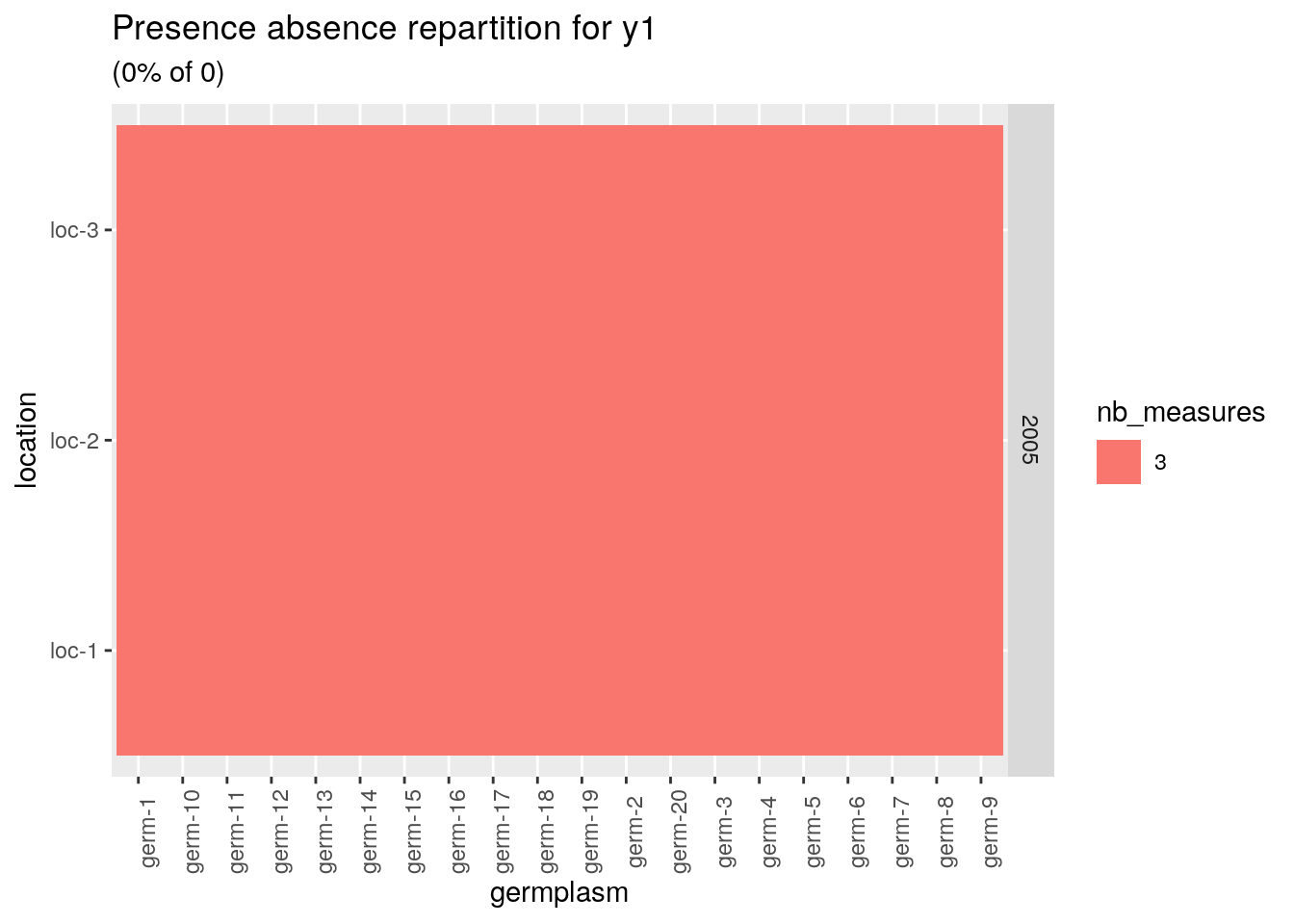or on the network with a pie with plot_type = "network" and by setting arguments data_to_pie and vec_variables:

# y1 is a quantitative variable
p_net_pies_y1 = plot(net_unipart_sl, data_to_pie, plot_type = "network", vec_variables = "y1")
p_net_pies_y1
## []
## []$y1_network_with_pies# y2 is a qualitative variable p_net_pies_y2 = plot(net_unipart_sl, data_to_pie, plot_type = "network", vec_variables = "y2") p_net_pies_y2 ## [] ## []$y2_network_with_pies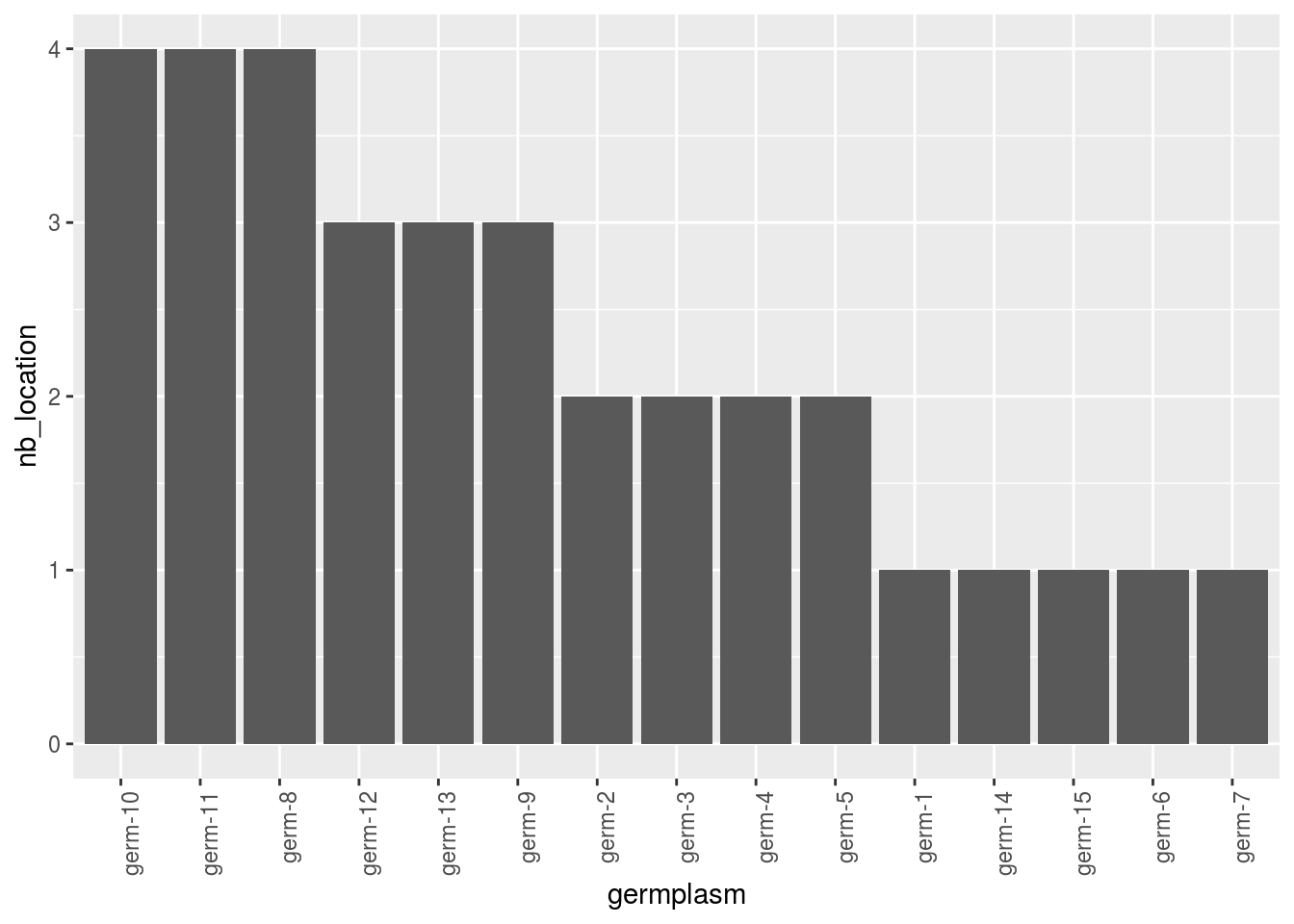The same can be done regarding relation type of the network on map but not on network.

p_map_pies_diff = plot.data_network(net_unipart_sl, plot_type = "map", vec_variables = "diffusion")
p_map_pies_diff
## []
## []$diffusion_nb_received_map_with_pies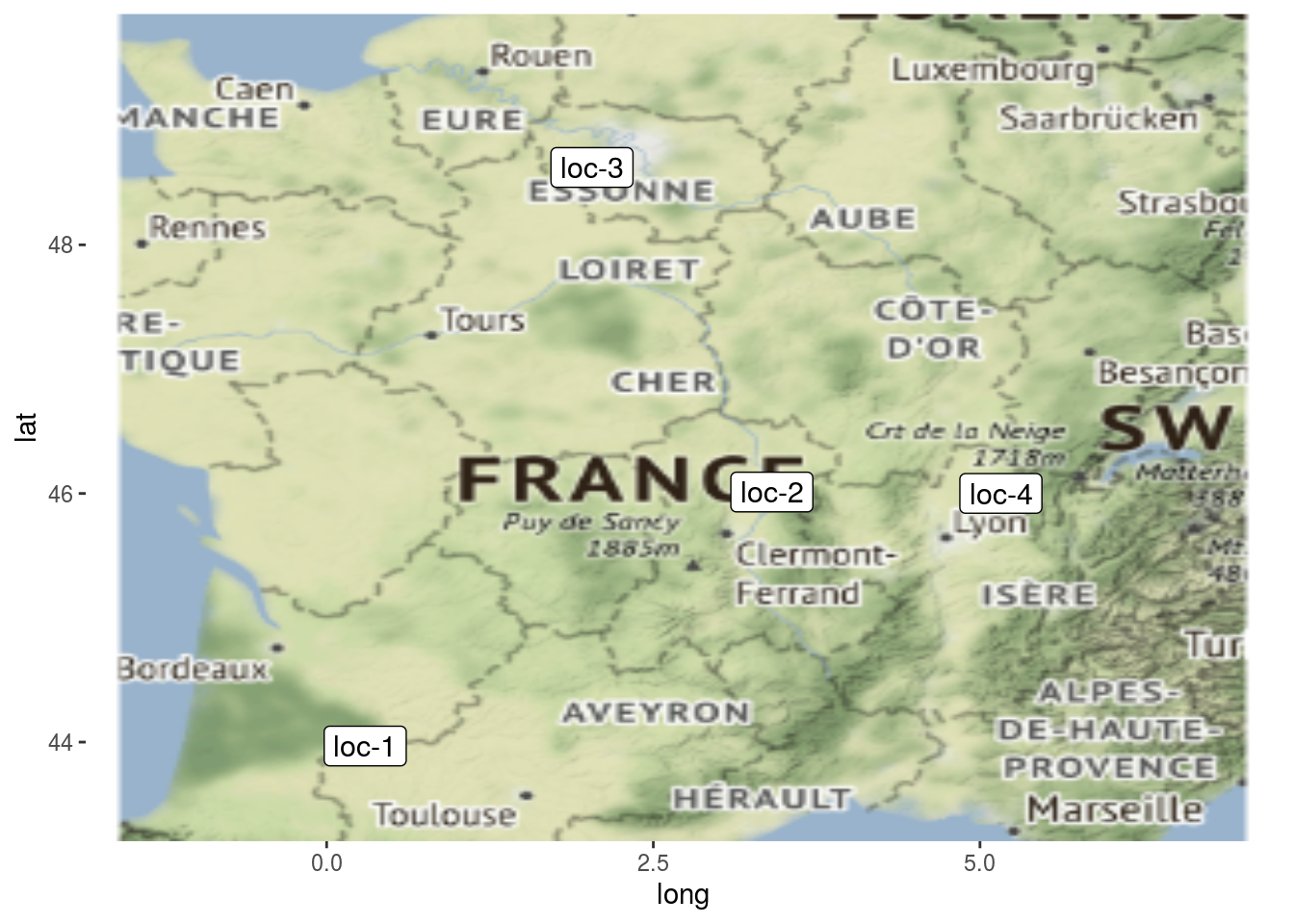## ## []$diffusion_nb_given_map_with_piesHere the pies represent the repartition of the number of seed lots.

### 2.2.2 unipart network on location

For network representation, set plot_type = "network" diffusion event are display with curve. in_col can be settle to customize color of vertex. The curve between location represent the diffusion, the number of diffusion is displayed on a color scale.

p_net = plot(net_unipart_location_g, plot_type = "network",
labels_on = "location", labels_size = 4)
names(p_net) # one element per germplasm, the first element with all the data
##  "germ-10-germ-11-germ-12-germ-13-germ-2-germ-3-germ-4-germ-5-germ-6-germ-7"
##  "germ-2"
##  "germ-3"
##  "germ-4"
##  "germ-5"
##  "germ-6"
##  "germ-7"
p_net$germ-2 ##$networkp_net = plot(net_unipart_location_y, plot_type = "network",
labels_on = "location", labels_size = 4)
names(p_net) # one element per year, the first element with all the data
##  "2007-2008-2009" "2007"           "2008"           "2009"
p_net$2007-2008-2009 ##$networkWith barplots, it represents the number of germplasm received or given.

p_bar = plot(net_unipart_location_y, plot_type = "barplot", x_axis = "location", in_col = "germplasm")
names(p_bar) # one element per year, the first element with all the data
##  "2007-2008-2009" "2007"           "2008"           "2009"
p_bar = p_bar$2007-2008-2009 p_bar$barplot$received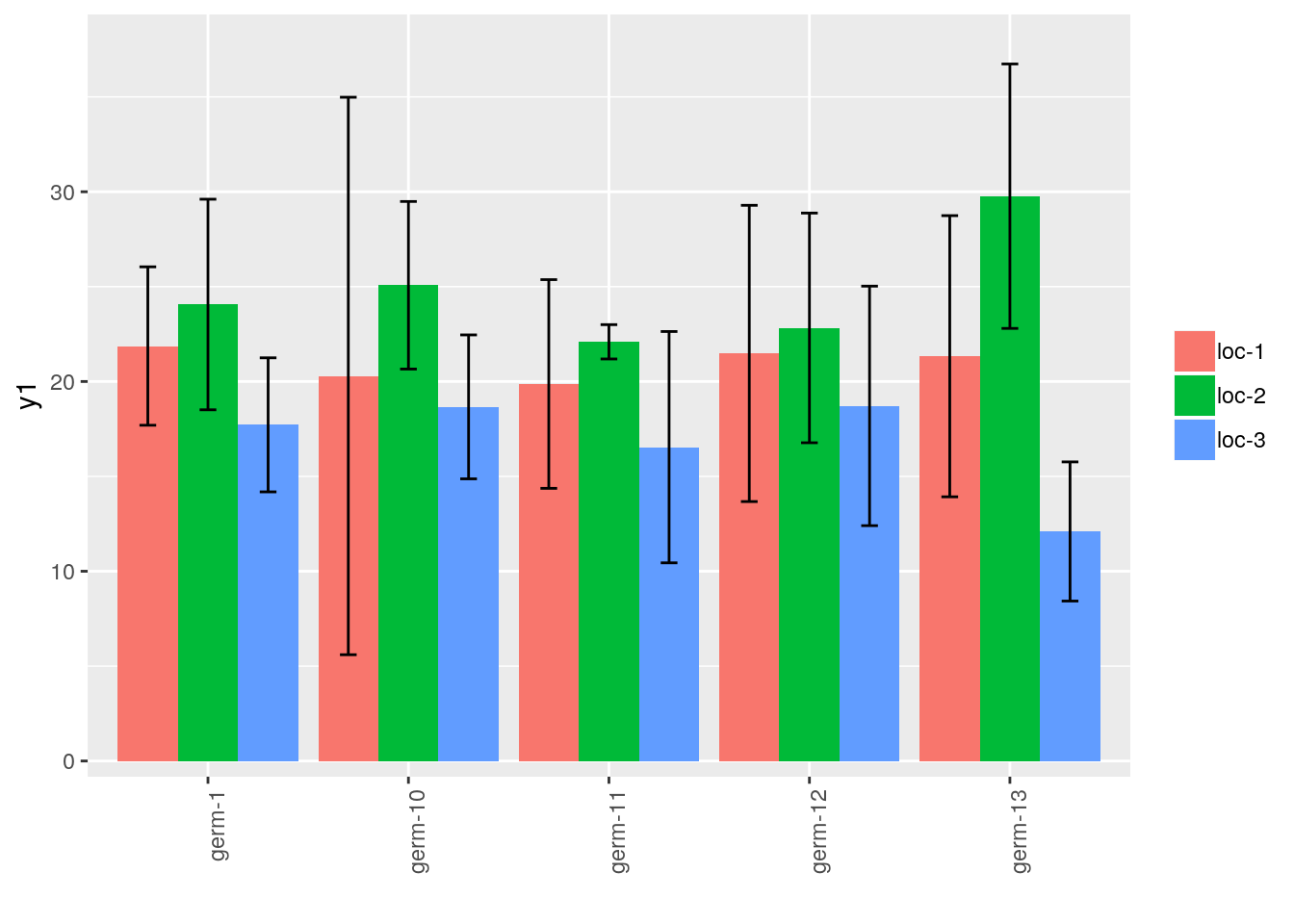p_bar$barplot$givenLocation present on the network can be displayed on a map with plot_type = "map". p_map = plot.data_network(net_unipart_location_y, plot_type = "map", labels_on = "location") # Note if you want to do it on all element of the list, you should use # plot(net_unipart_location_y, plot_type = "map", labels_on = "location") # Here we use plot.data_network only not to ask to often the map server that may bug if there # are too many query p_map$2007-2008-2009
## $map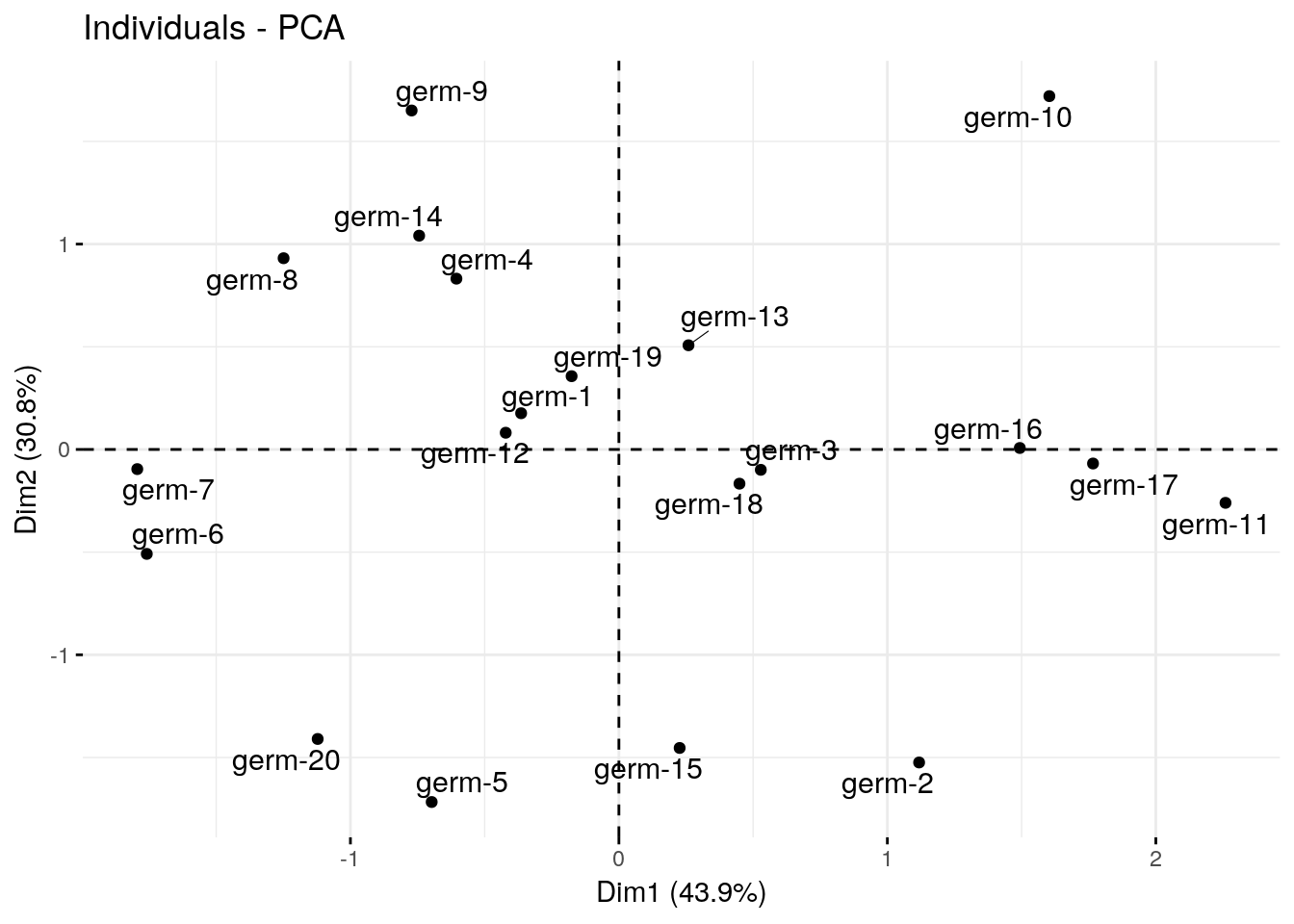As well as plot information regarding a variable on map with a pie with plot_type = "map" and by setting arguments data_to_pie and vec_variables: # y1 is a quantitative variable p_map_pies_y1 = plot.data_network(net_unipart_location_y, data_to_pie, plot_type = "map", vec_variables = "y1") p_map_pies_y1$2007-2008-2009
## list()
# y2 is a qualitative variable
p_map_pies_y2 = plot.data_network(net_unipart_location_y, data_to_pie, plot_type = "map", vec_variables = "y2")
p_map_pies_y2$2007-2008-2009 ## list() Note that it is not possible to display plot with plot_type = "network". ### 2.2.3 bipart network on germplasm and location p_net = plot(net_bipart, plot_type = "network", labels_on = TRUE, labels_size = 4) names(p_net) # one element per year, the first element with all the data ##  "2005-2006-2007-2008-2009" "2005" ##  "2006" "2007" ##  "2008" "2009" p_net$2009
## $network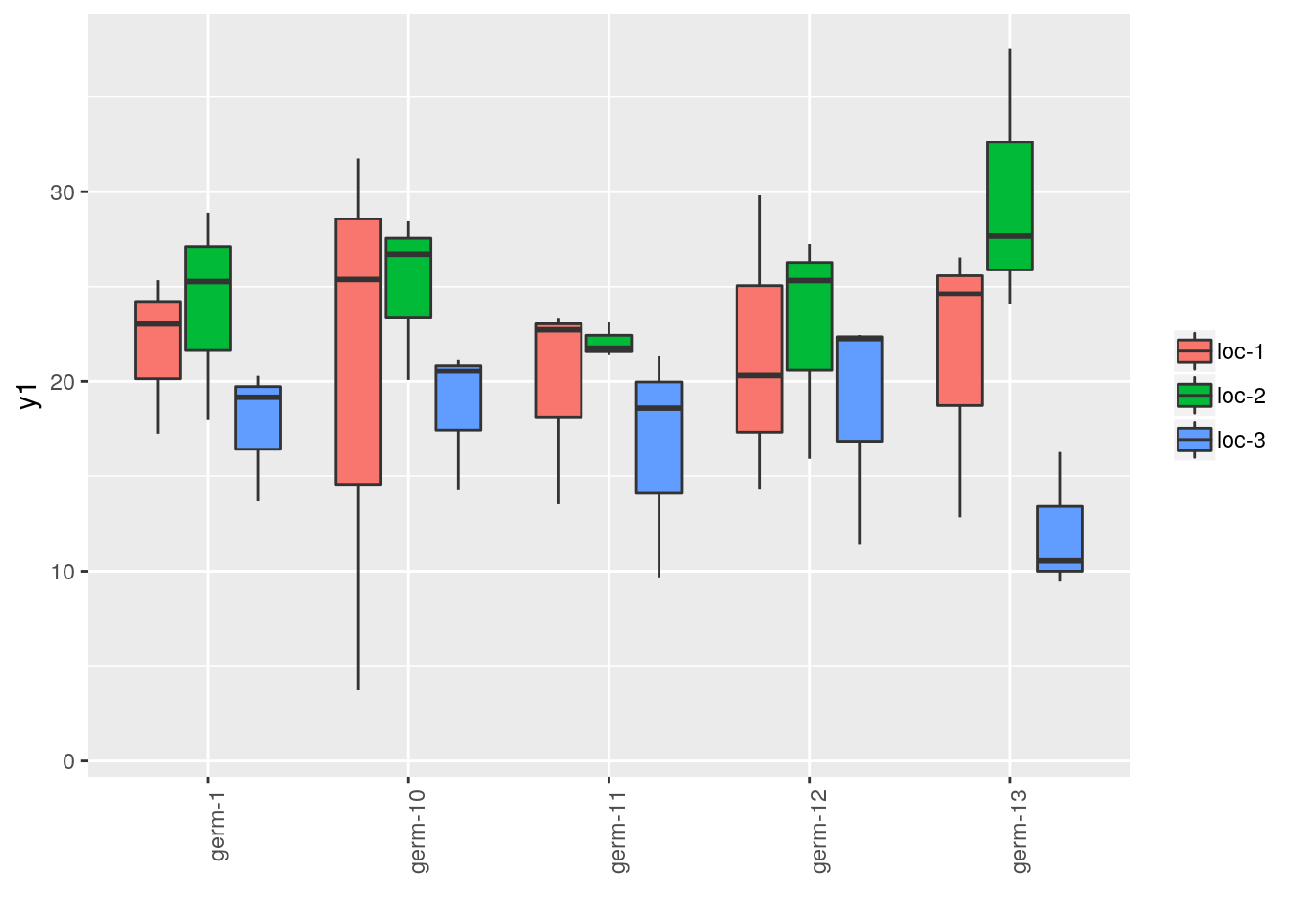With barplots, it represents the number of edges per vertex for each germplasm and each location. p_bar = plot(net_bipart, plot_type = "barplot") names(p_bar) # one element per year, the first element with all the data ##  "2005-2006-2007-2008-2009" "2005" ##  "2006" "2007" ##  "2008" "2009" p_bar = p_bar$2005-2006-2007-2008-2009$barplot p_bar$germplasm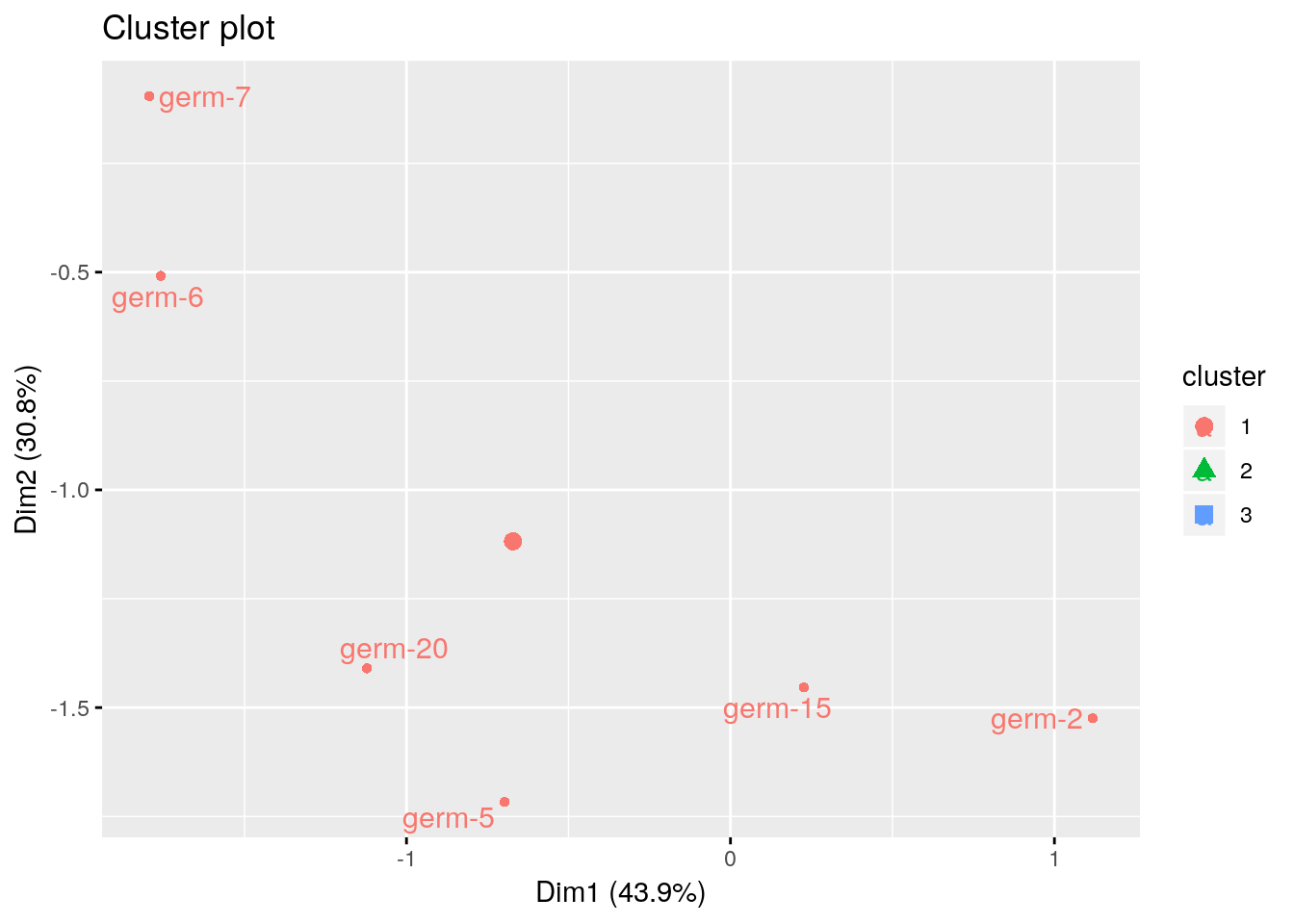p_bar$locationLocation present on the network can be displayed on a map with plot_type = "map". p_map = plot.data_network(net_bipart, plot_type = "map", labels_on = "location") p_map$2005-2006-2007-2008-2009
## $map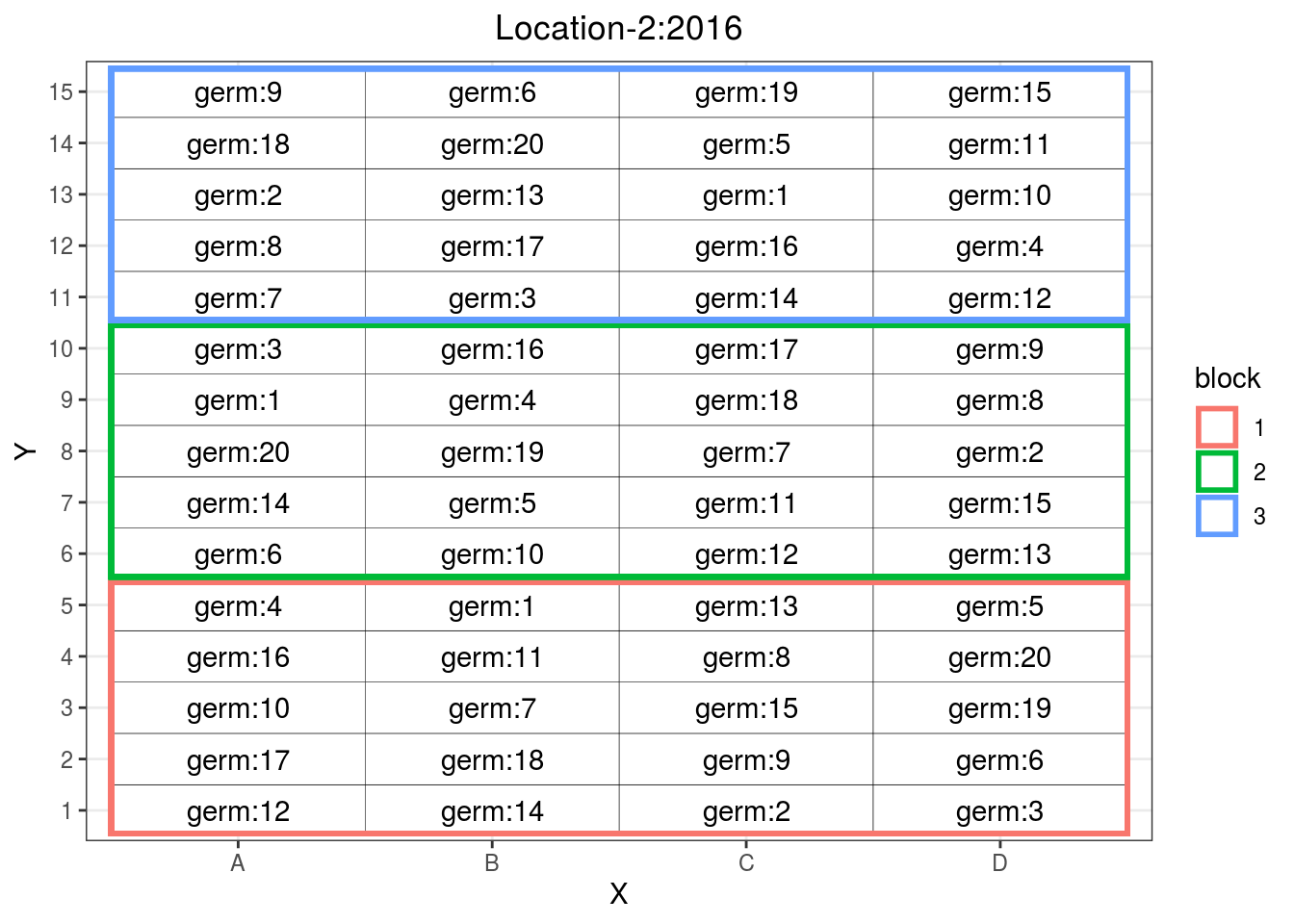As well as plot information regarding a variable on map with a pie with plot_type = "map" and by setting arguments data_to_pie and vec_variables: # y1 is a quantitative variable p_map_pies_y1 = plot.data_network(net_bipart, data_to_pie, plot_type = "map", vec_variables = "y1") p_map_pies_y1$2005-2006-2007-2008-2009
## $y1_map_with_pies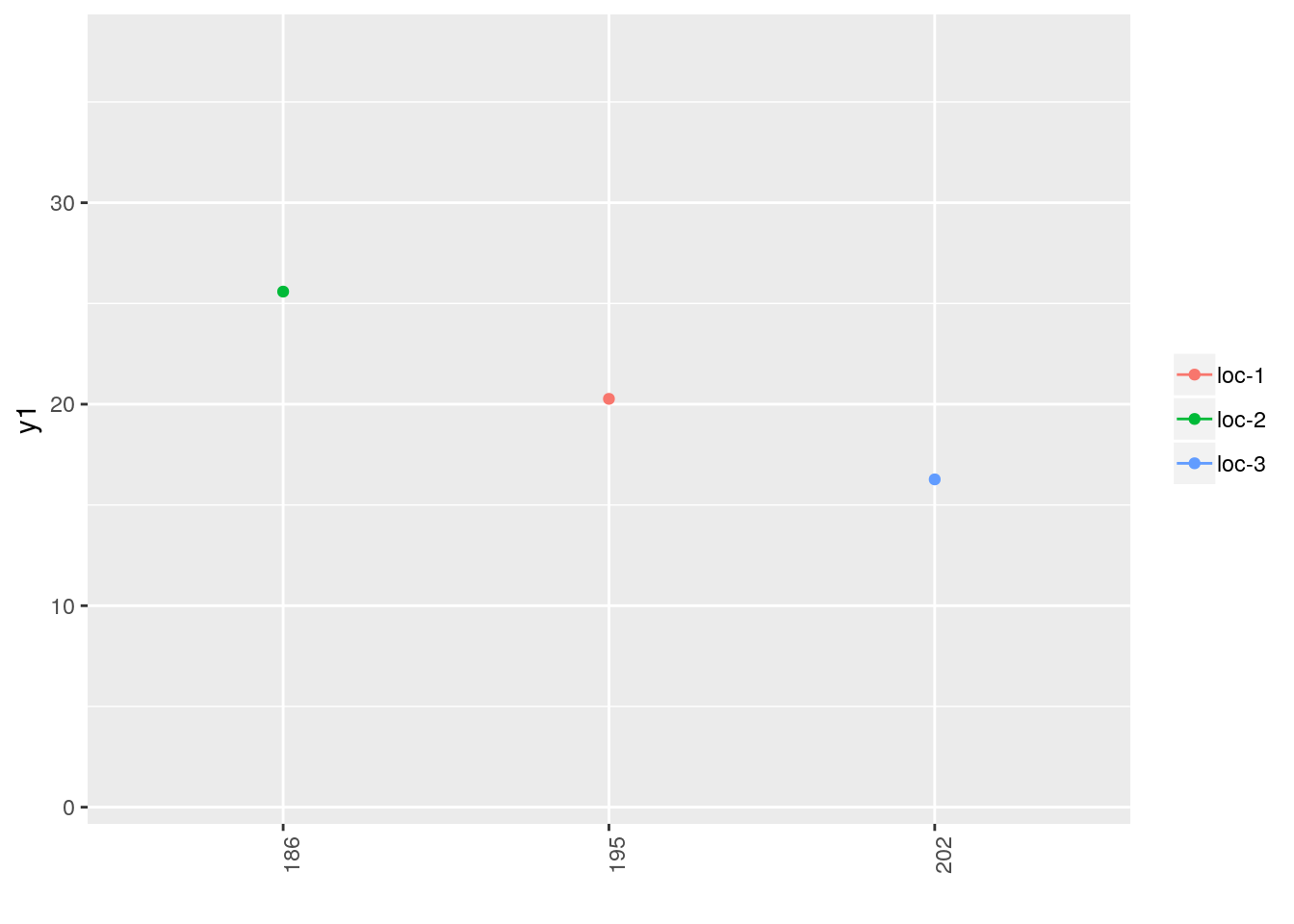# y2 is a qualitative variable p_map_pies_y2 = plot.data_network(net_bipart, data_to_pie, plot_type = "map", vec_variables = "y2") p_map_pies_y2$2005-2006-2007-2008-2009
## \$y2_map_with_piesNote that it is not possible to display plot with plot_type = "network".